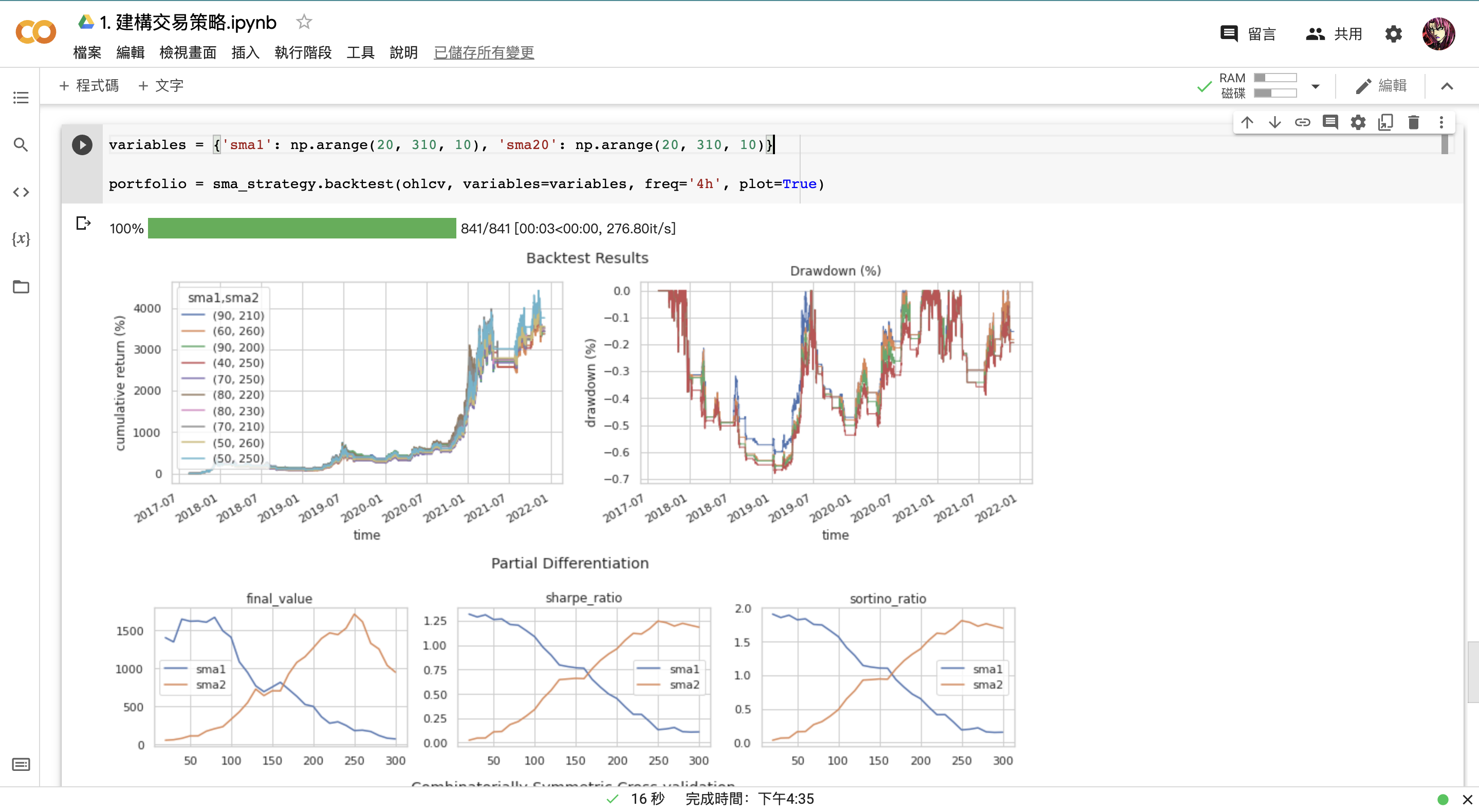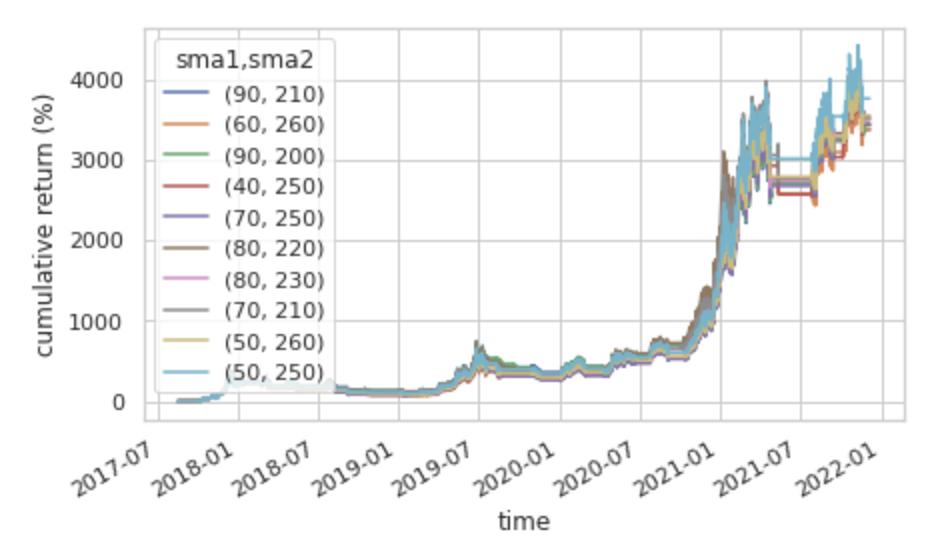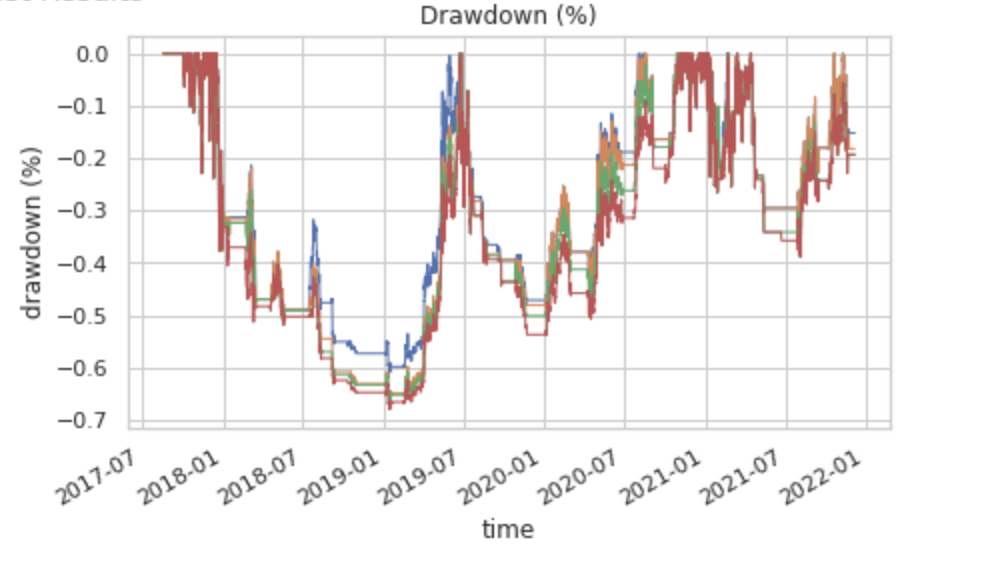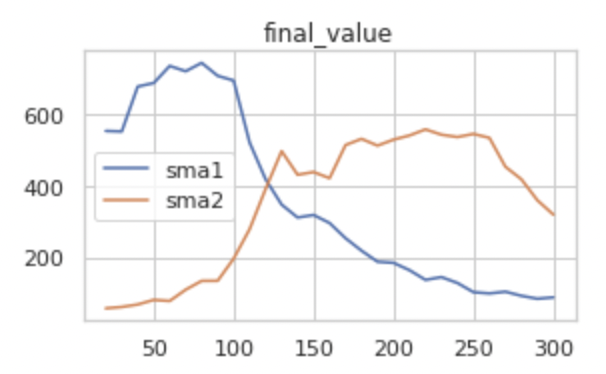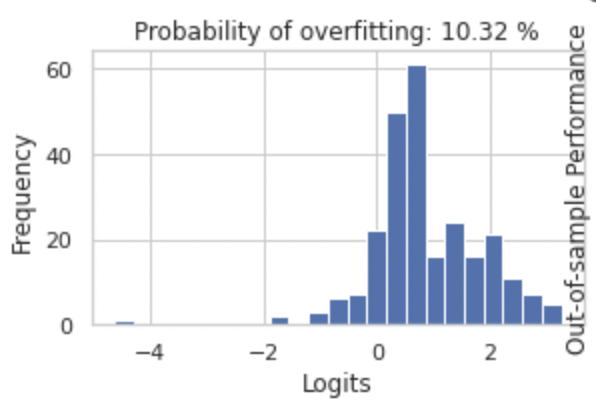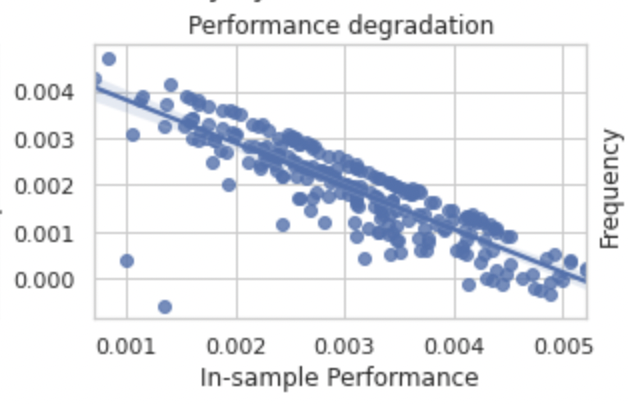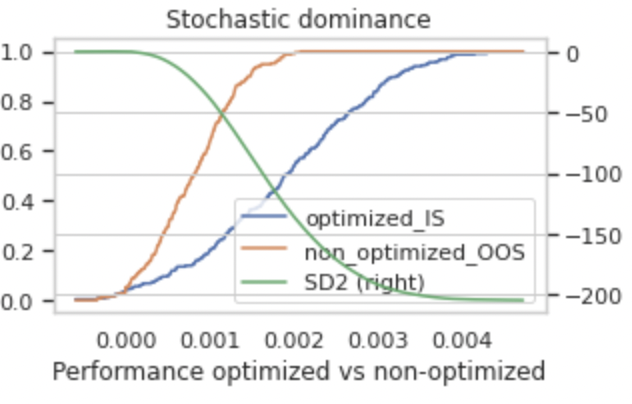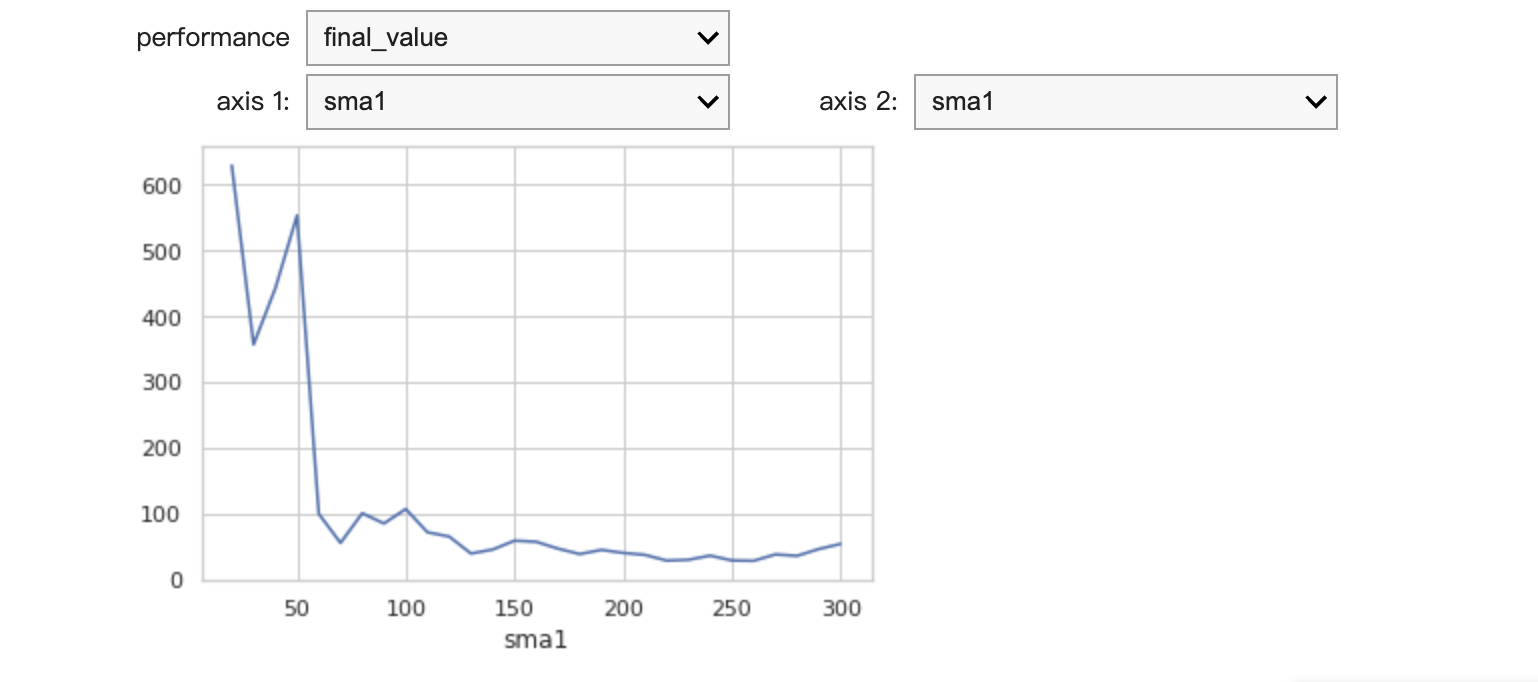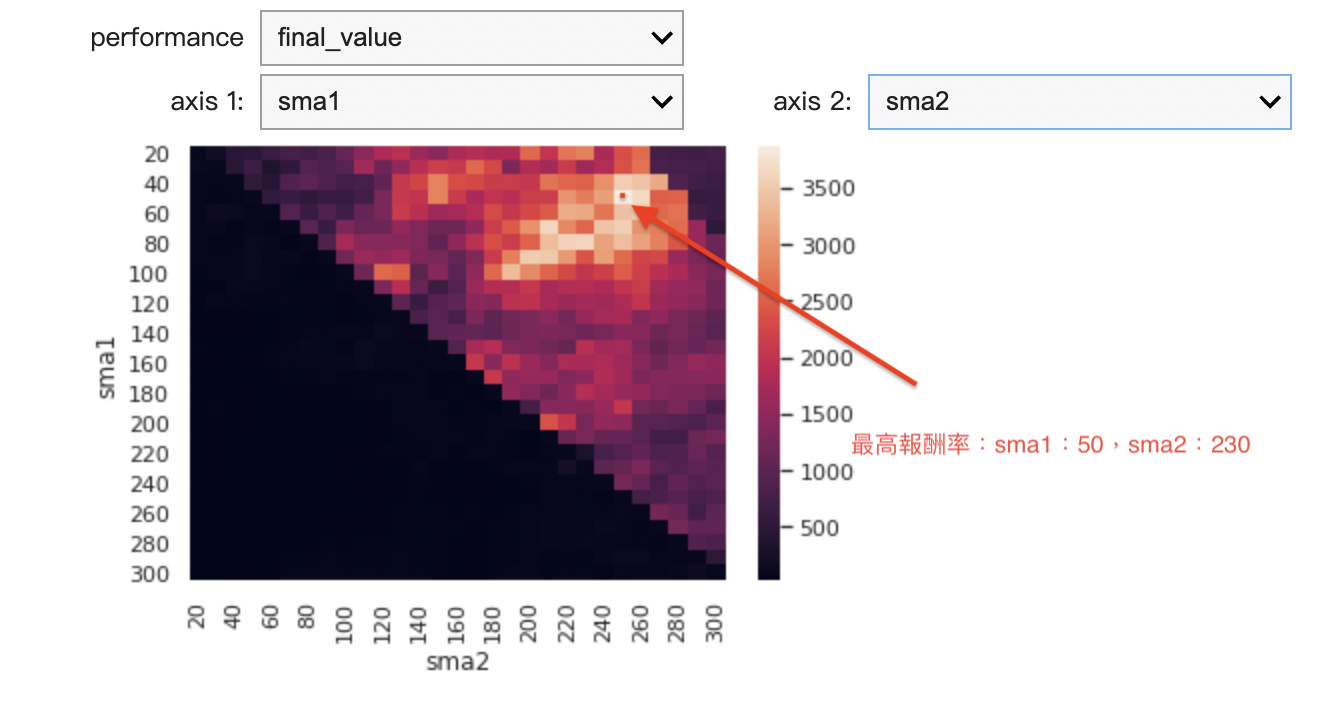# 第一章：5 找出策略最佳參數

### a. 參數設定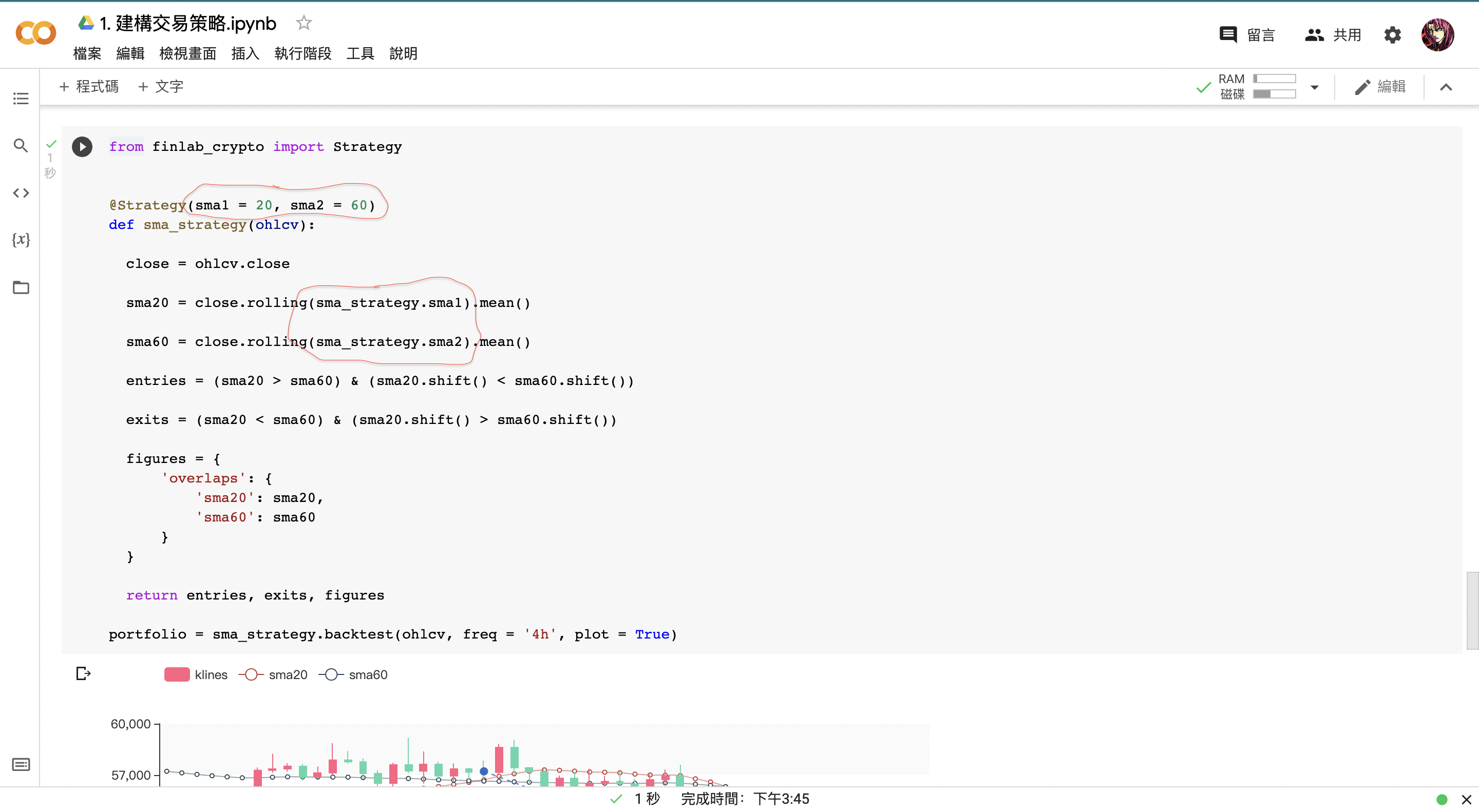portfolio = sma_strategy.backtest(ohlcv, variables = {'sma1': 20, 'sma20': 60}, freq = '4h', plot = True)


portfolio = sma_strategy.backtest(ohlcv, variables = variables, freq = '4h', plot = True)


### b. 參數配對

import numpy as np


np.arange(20, 310, 10)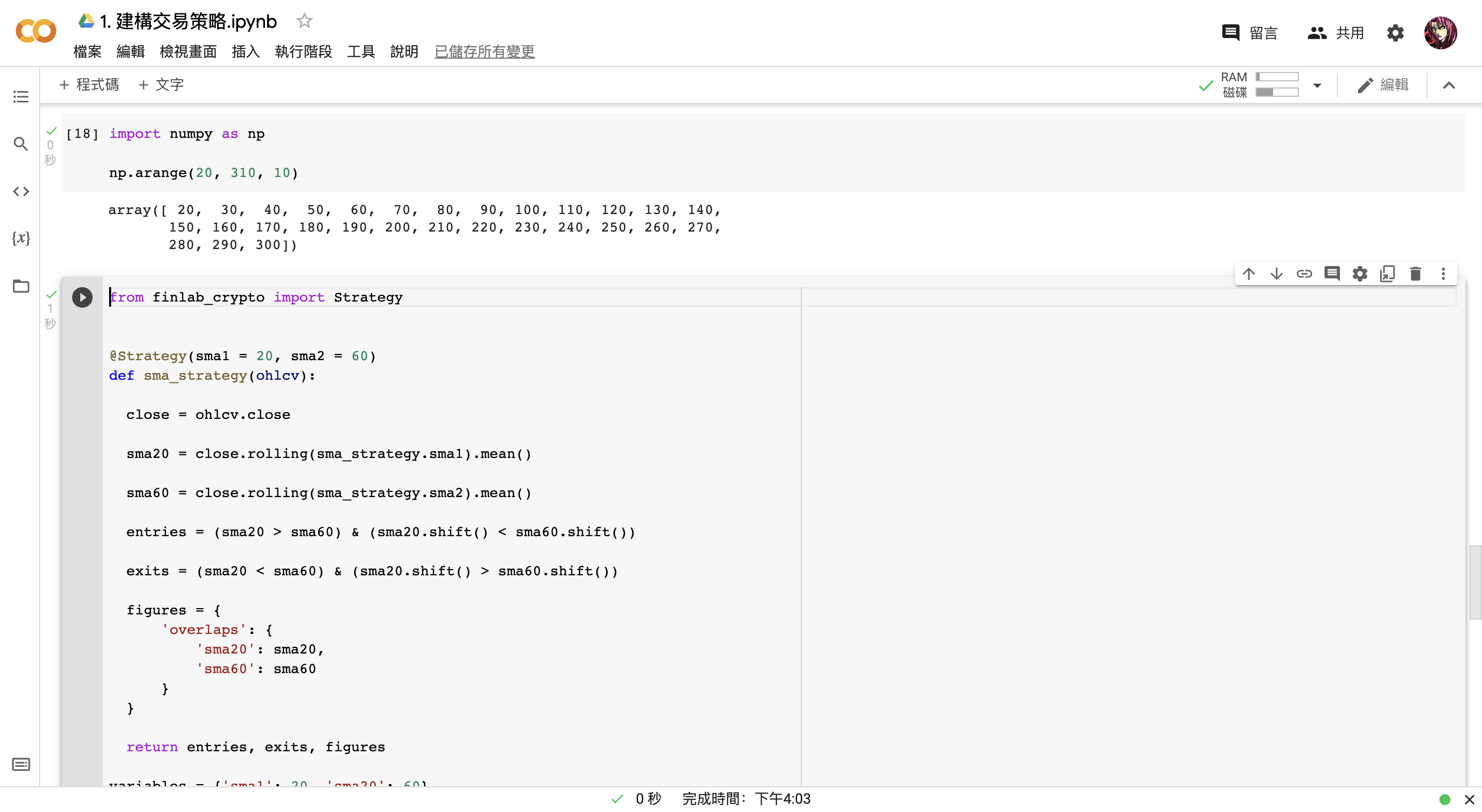variables = {'sma1': np.arange(20, 310, 10), 'sma2': np.arange(20, 310, 10)}